# NCERT Solutions for Class 8 Maths Chapter 9 Exercise 9.2 – Algebraic Expressions & Identities

NCERT Solutions for Class 8 Maths Chapter 9 Exercise 9.2 – Algebraic Expressions & Identities, has been designed by the NCERT to test the knowledge of the student on the following topics:-

• Multiplication of Algebraic Expressions: Introduction
• Multiplying a Monomial by a Monomial
– Multiplying two monomials
– Multiplying three or more monomials

### NCERT Solutions for Class 8 Maths Chapter 9 Exercise 9.2 – Algebraic Expressions & Identities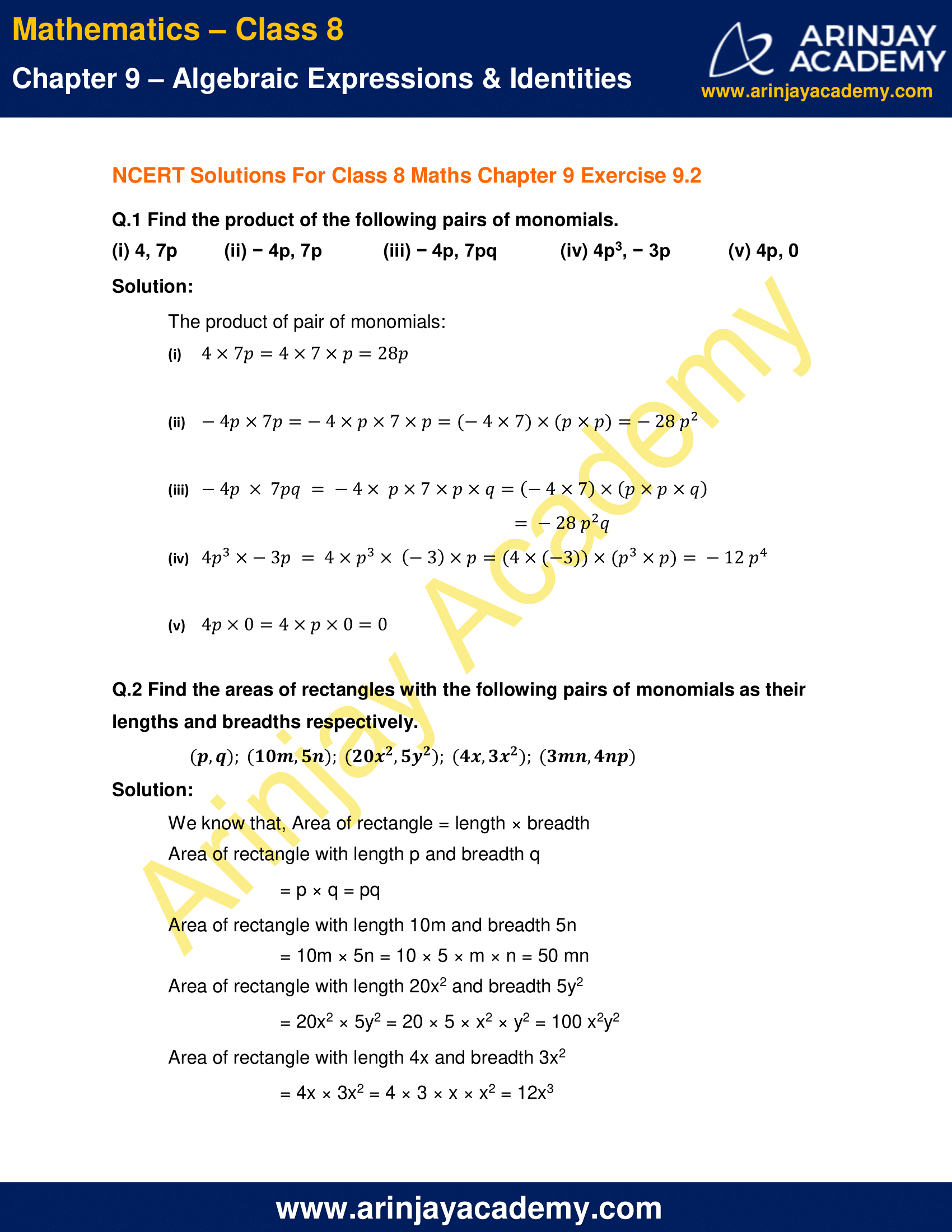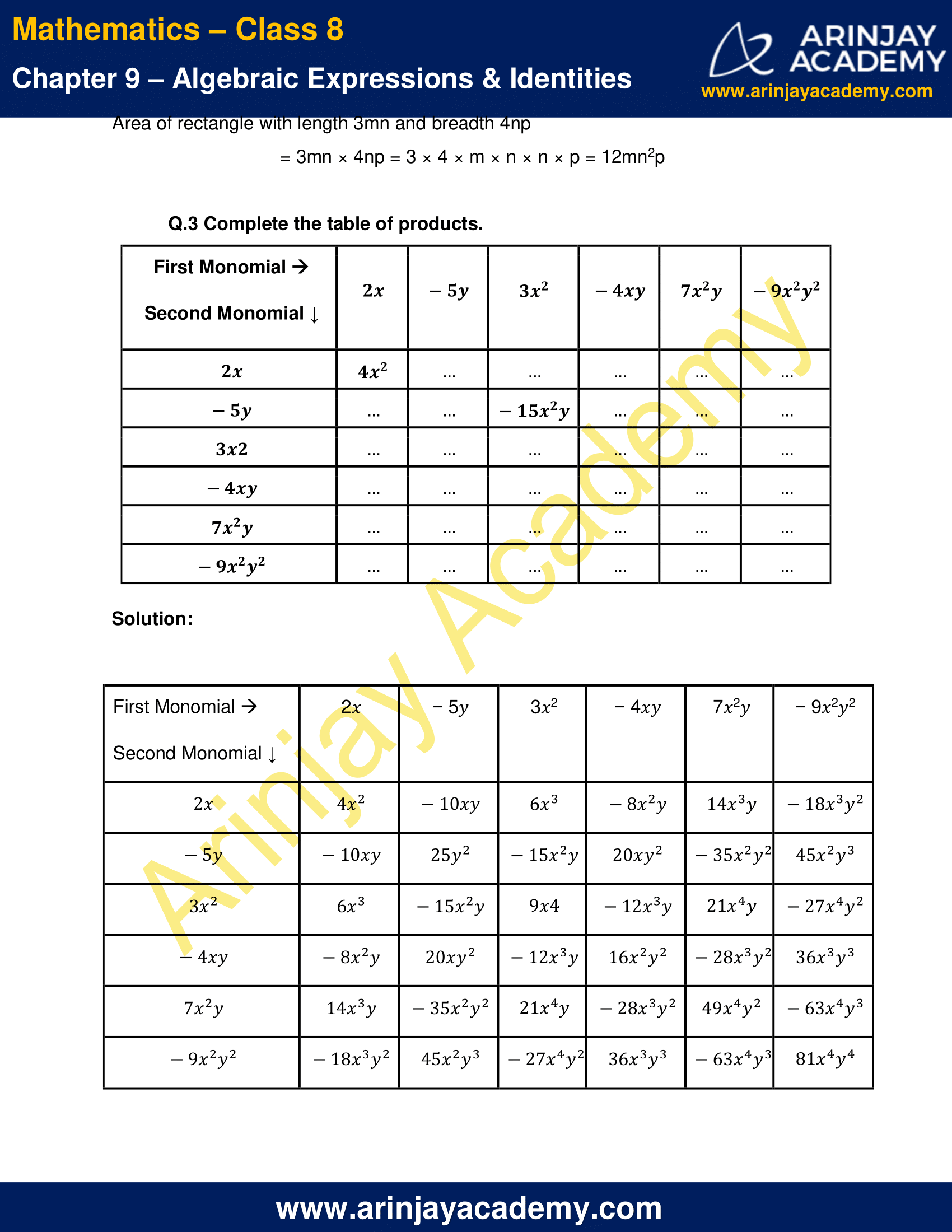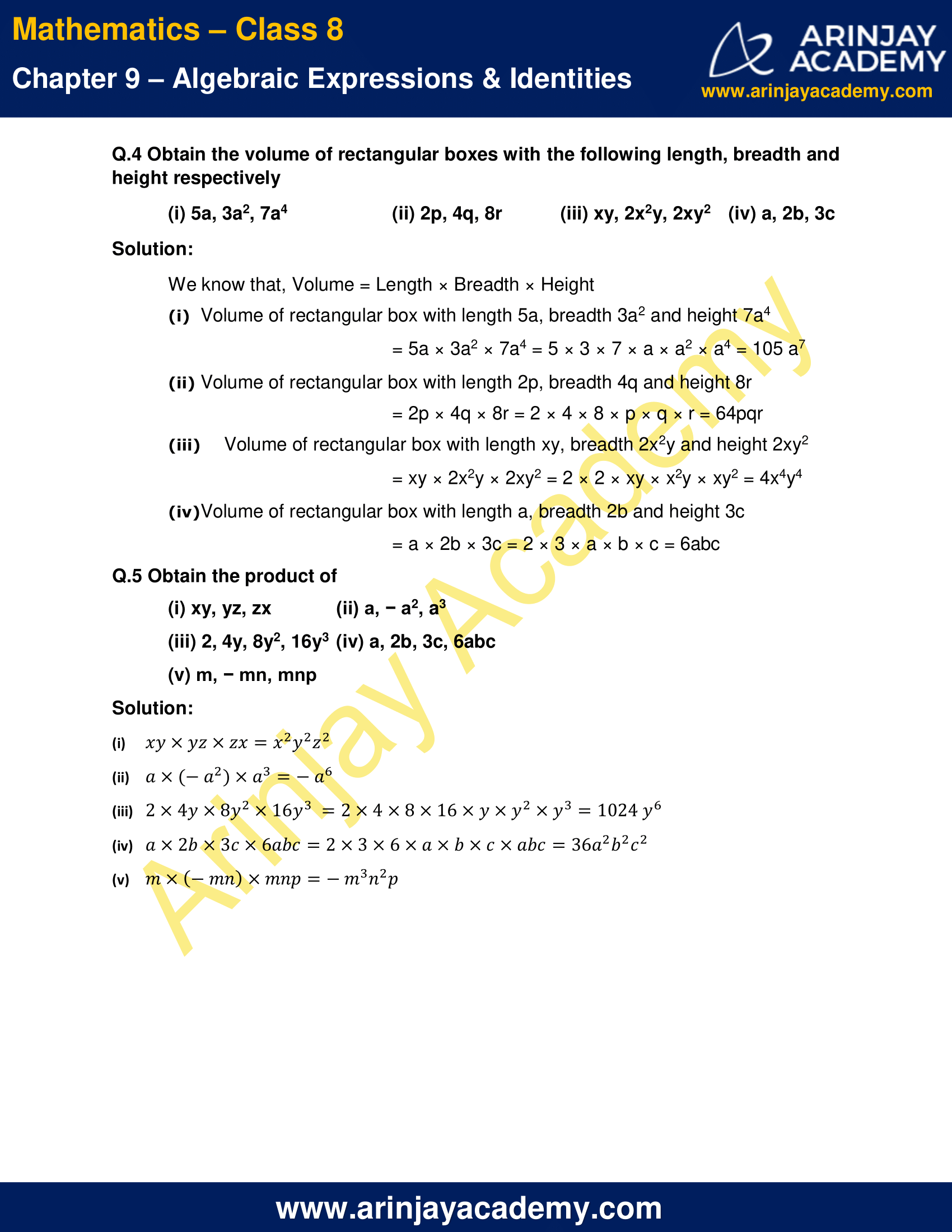NCERT Solutions for Class 8 Maths Chapter 9 Exercise 9.2 – Algebraic Expressions & Identities

Q.1 Find the product of the following pairs of monomials.

(i) 4, 7p
(ii) − 4p, 7p
(iii) − 4p, 7pq
(iv) 4p3, − 3p
(v) 4p, 0

Solution:

The product of pair of monomials: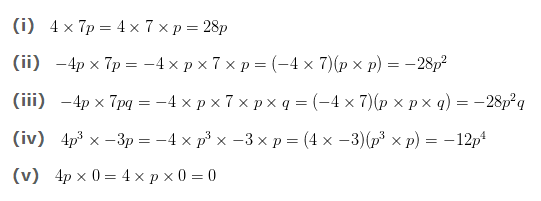Q.2 Find the areas of rectangles with the following pairs of monomials as their lengths and breadths respectively.

(p,q); (10m, 5n); (20x², 5y²); (4x, 3x²); (3mn, 4np)

Solution:

We know that, Area of rectangle = length × breadth

Area of rectangle with length p and breadth q

= p × q = pq

Area of rectangle with length 10m and breadth 5n

= 10m × 5n = 10 × 5 × m × n = 50 mn

Area of rectangle with length 20x2 and breadth 5y2

= 20x2 × 5y2 = 20 × 5 × x2 × y2 = 100 x2y2

Area of rectangle with length 4x and breadth 3x2

= 4x × 3x2 = 4 × 3 × x × x2 = 12x3

Area of rectangle with length 3mn and breadth 4np

= 3mn × 4np = 3 × 4 × m × n × n × p = 12mn2p

Q.3 Complete the table of products.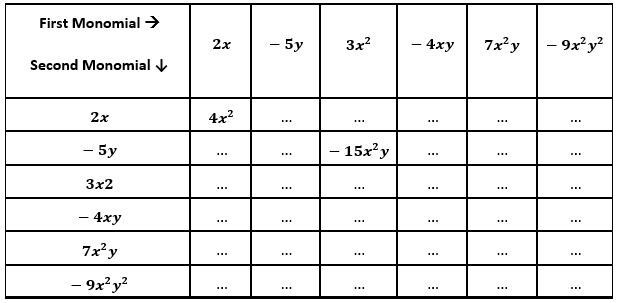Solution: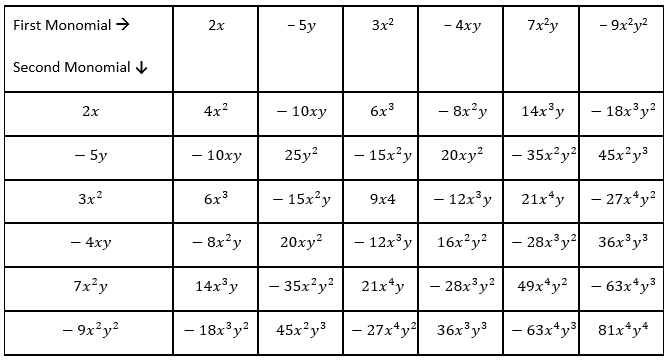Q.4 Obtain the volume of rectangular boxes with the following length, breadth and height respectively

(i) 5a, 3a2, 7a4
(ii) 2p, 4q, 8r
(iii) xy, 2x2y, 2xy2
(iv) a, 2b, 3c

Solution:

We know that, Volume = Length × Breadth × Height

(i) Volume of rectangular box with length 5a, breadth 3a2 and height 7a4

= 5a × 3a2 × 7a4 = 5 × 3 × 7 × a × a2 × a4 = 105 a7

(ii) Volume of rectangular box with length 2p, breadth 4q and height 8r

= 2p × 4q × 8r = 2 × 4 × 8 × p × q × r = 64pqr

(iii) Volume of rectangular box with length xy, breadth 2x2y and height 2xy2

= xy × 2x2y × 2xy2 = 2 × 2 × xy × x2y × xy2 = 4x4y4

(iv) Volume of rectangular box with length a, breadth 2b and height 3c

= a × 2b × 3c = 2 × 3 × a × b × c = 6abc

Q.5 Obtain the product of

(i) xy, yz, zx
(ii) a, − a2, a3
(iii) 2, 4y, 8y2, 16y3
(iv) a, 2b, 3c, 6abc
(v) m, − mn, mnp

Solution: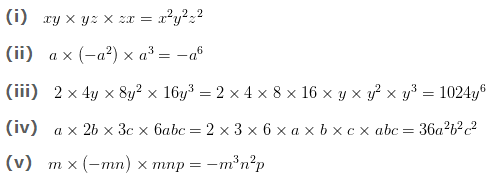The next Exercise for NCERT Solutions for Class 8 Maths Chapter 9 Exercise 9.3 – Algebraic Expressions and Identities can be accessed by clicking here

NCERT Solutions For Class 8 Maths Chapter 9 Exercise 9.2 – Algebraic Expressions & Identities